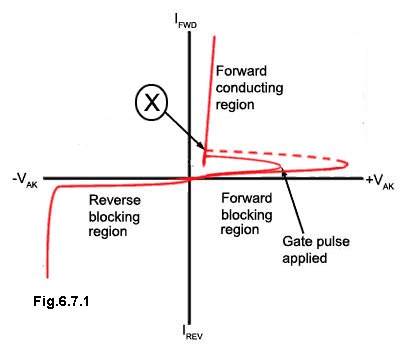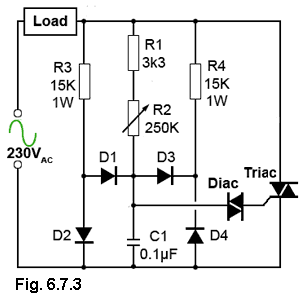# Thyristor Quiz

Try this quiz based on thyristors. Hopefully it'll be easy. Submit your answers but don't be disappointed if you get answers wrong. All the information you need is on this website. Find the right answer and learn about thyristors as you go.

### 1.What is the value indicated by point X in Fig. 6.7.1?

### 2.

What is the function of a crowbar protection circuit?

### 3.Referring to Fig. 6.7.2, what is the maximum achievable conduction angle using this circuit?

### 4.

Which of the following advantages and disadvantages describes a zero crossing SCR circuit?

### 5.

Which quadrants are used when triggering a triac with a negative going trigger pulse in both the positive and negative half cycles of the AC wave?

### 6.Referring to Fig. 6.7.3, what is the purpose of diodes D1 to D4 in this circuit?

### 7.

What is the function of a Metal Oxide Varistor (MOV) in the protection of thyristor power supplies?

### 8.

Under which of the following conditions will a triac cease conducting?

### 9.

Which of the following statements is untrue?

### 10.

What is the function of a TVS diode when used in a high power SSR?